Previous: Algorithms PISO and SIMPLE Up: Algorithms PISO and SIMPLE Next: SIMPLE

This is an automatically generated documentation by LaTeX2HTML utility. In case of any issue, please, contact us at info@cfdsupport.com.

## PISO

• Assume Navier-Stokes equations for incompressible fluid flow
• Mass conservation equation (continuity equation)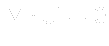(11.1)

• Momentum conservation equation (velocity equation)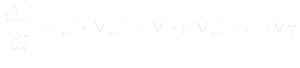(11.2)
• When discretized momentum conservation equation: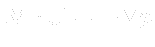(11.3)
• Matrix linear system: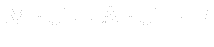(11.4)
• Matrix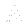is diagonal, with diagonal of original matrix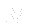•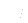is vector corresponding to ()
• Rewriting () with substitution from ():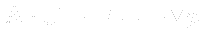(11.5)

• Express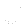from equation (), i.e. momentum correction equation :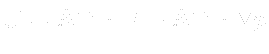(11.6)• We combine momentum correction equation () and continuity equation (), which gives us: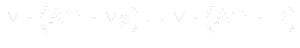(11.7)
• PISO algorithm description:
1. Computation starts, initialand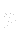are chosen
2. Time step starts
3. Boundary conditions are updated
4. Linear system is solved5. Obtained new6. Building matrixand vector7. Mass flow over cell faces is computed
8. Pressure correction is applied ()
9. Obtained new10. Mass flow over cell faces is corrected
11. Momentum correction is applied ()
12. Obtained new corrected13. Boundary conditions are updated
14. Steps 6 – 13 are repeated with respect to nCorrectors value
15. Time step ends
• PISO algorithm in source code:
• Take a look at solver icoFoam:
# less \$FOAM_SOLVERS/incompressible/icoFoam/icoFoam.C
``` Info<< "\nStarting time loop\n" << endl;
```
• At the beginning of the computation the output is : Starting time loop
```    while (runTime.loop())
{
Info<< "Time = " << runTime.timeName() << nl << endl;

#include "CourantNo.H"
```
• Cycle over all time steps
• Actual time layer is written on the output
```        fvVectorMatrix UEqn
(
fvm::ddt(U)
+ fvm::div(phi, U)
- fvm::laplacian(nu, U)
);
```
• Momentum matrixis created (UEqn)
• UEqn is the left hand side of equation ()
```        solve(UEqn == -fvc::grad(p));
```
• Solves system```        // --- PISO loop

for (int corr=0; corr<nCorr; corr++)
{
```
• Start of PISO algorithm
• Loop runs until the repetition reaches the value nCorrectors
```            volScalarField rAU(1.0/UEqn.A());
```
• The matrix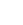is saved as rAU
```            volVectorField HbyA(constrainHbyA(rAU*UEqn.H(), U, p));
```
• Evaluates relation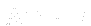and saves to Hbya
```           surfaceScalarField phiHbyA
(
"phiHbyA",
fvc::flux(HbyA)
+ fvc::interpolate(rAU)*fvc::ddtCorr(U, phi)
);

// Update the pressure BCs to ensure flux consistency
constrainPressure(p, U, phiHbyA, rAU);

// Non-orthogonal pressure corrector loop
while (piso.correctNonOrthogonal())
{
// Pressure corrector

fvScalarMatrix pEqn
(
fvm::laplacian(rAU, p) == fvc::div(phiHbyA)
);

pEqn.setReference(pRefCell, pRefValue);

pEqn.solve(mesh.solver(p.select(piso.finalInnerIter())));
```
• Pressure correction loop solves equation```                if (piso.finalNonOrthogonalIter())
{
phi = phiHbyA - pEqn.flux();
}
}
```
• Momentum correction
```            #include "continuityErrs.H"
```
• Including header file for continuity error evaluation
```            U = HbyA - rAU*fvc::grad(p);
U.correctBoundaryConditions();
}
```
• Momentum (velocity) correction
• Boundary conditions correction
• End of current time step
```        runTime.write();
```
• Writes data to disk (if it is in the time layer where it is told to write down)
```        Info<< "ExecutionTime = " << runTime.elapsedCpuTime() << " s"
<< "  ClockTime = " << runTime.elapsedClockTime() << " s"
<< nl << endl;
}

Info<< "End\n" << endl;
```
• Writes time information
• End of computation